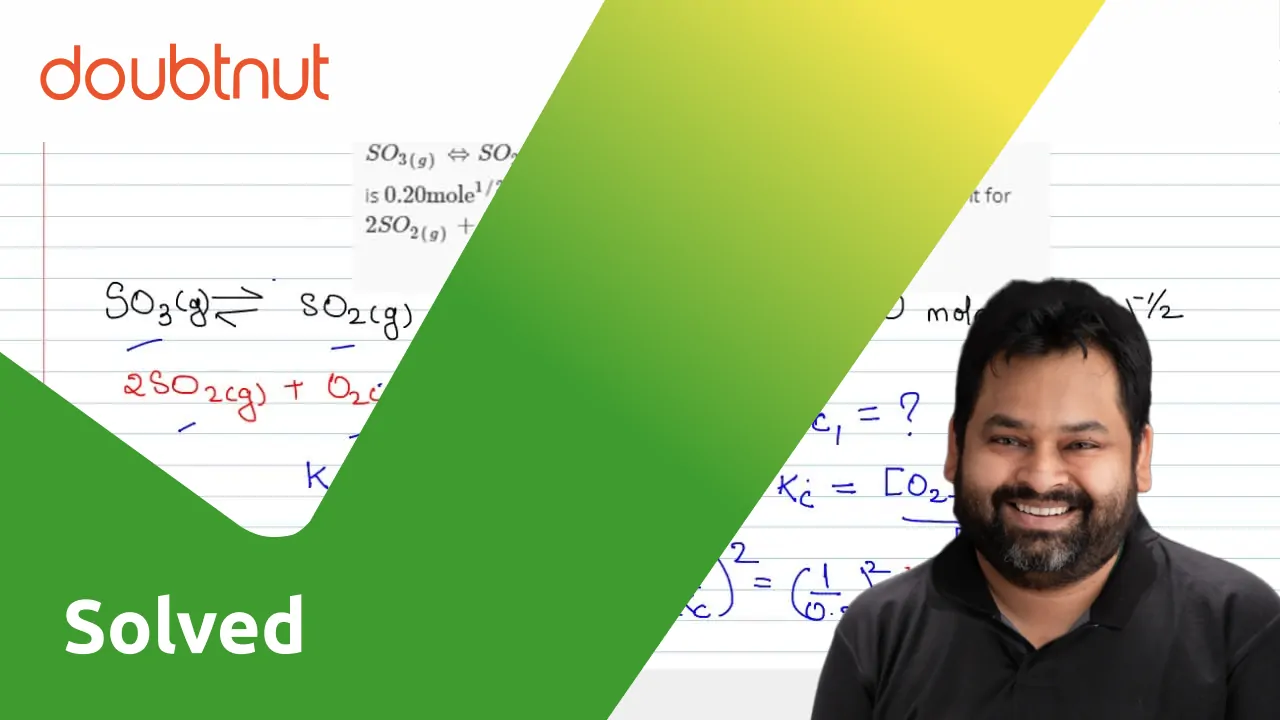Doubtnut App - Get 50 Lakh+ video solutions better than GoogleInstall Now

# The equilibrium constant of the reaction , SO3(g)⇔SO2(g)+12O2(g), is 0.20mole1/2litre−1/2 at 1000K. Calculate equilibrium constant for 2SO2(g)+O2(g)⇔2SO3(g)

Text Solution
Verified by Experts

## For SO3(g)⇔SO2(g)+12O2(g) Kc1=[SO2][O2]1/2[SO3]=0.20 …(1) For 2SO2+O2⇔2SO3 Kc=[SO3]2[SO2]2[O2] …(2) By reversing eq. (1), 1Kc1=[SO3][SO2][O2]1/2 Squaring both sides by eq. (2), (1Kc1)=[SO3]2[SO2]2[O2]=Kc ∴Kc=[10.20]2=25mol−1litre+1